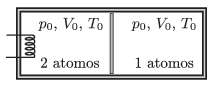Mathematical and Physical Journal
for High Schools
Issued by the MATFUND Foundation
 Already signed up? New to KöMaL?

#Problem P. 4678. (November 2014)

P. 4678. A frictionlessly moving piston, which has negligible heat capacity and which is made of some heat conducting material, divides a thermally insulated container into two parts. Initially the chamber at the left contains a sample of diatomic gas and in the chamber at the right there is a sample of monatomic gas, such that the two samples of gas have the same $\displaystyle V_0$ volume, the same $\displaystyle p_0$ pressure and also the same $\displaystyle T_0$ temperature. There is an electric heating element in the left chamber and thermal energy of $\displaystyle Q$ is added to the gas. Due to the added heat 20% of the molecules of the diatomic gas dissociate to monatomic particles. The energy needed for the dissociation of one mole gas is $\displaystyle D$.After thermal equilibrium is reached what will the volume of the gas in the left chamber be, and what will its temperature be?

Data: $\displaystyle p_0=10^5$ Pa, $\displaystyle V_0=3.3$ liter, $\displaystyle T_0=300$ K, $\displaystyle Q=15.6$ kJ és $\displaystyle D=3\cdot10^5$ J/mol.

(5 pont)

Deadline expired on December 10, 2014.

### Statistics:

 58 students sent a solution. 5 points: Alabér Ádám, Ardai István Tamás, Asztalos Bogdán, Balogh Menyhért, Berczi Benjámin, Blum Balázs, Bugár 123 Dávid, Csathó Botond, Csorba Benjámin, Di Giovanni Márk, Fehér Balázs, Fekete Panna, Forrai Botond, Fülöp Erik, Holczer András, Iván Balázs, Jakus Balázs István, Jeges Viktor, Kaposvári Péter, Kasza Bence, Koch Lilla, Kovács Péter Tamás, Körtefái Dóra, Marosvári Kristóf, Németh Flóra Boróka, Olosz Balázs, Orosz Bálint, Öreg Botond, Plaszkó Noel, Rózsa Tibor, Sal Kristóf, Szántó Benedek, Szász Norbert Csaba, Szentivánszki Soma , Szépfalvi Bálint, Tanner Martin, Tibay Álmos, Tompa Tamás Lajos, Trócsányi Péter, Varga-Umbrich Eszter, Vastag Balázs, Wiandt Péter. 4 points: Berta Dénes, Büki Máté, Farkas 96 Vajk, Kormányos Hanna Rebeka, Mándoki László, Németh 017 András, Varju Ákos. 3 points: 2 students. 2 points: 3 students. 1 point: 3 students. 0 point: 1 student.

Problems in Physics of KöMaL, November 2014